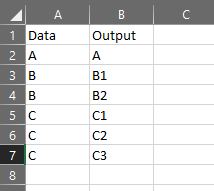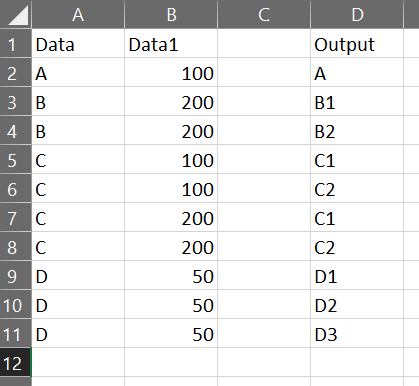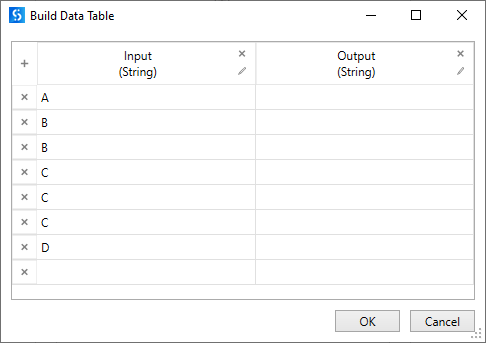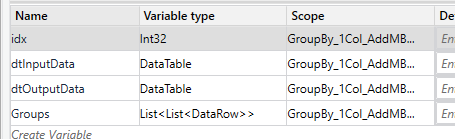# Increase same valued data consecutively if occurs more than once

Suppose I’ve a dataset where same data can occur more than once. If that happens I want to increase the value consecutively. I’m adding sample data and the output.But I’m unable to solve it. Need some help here as I’m still learning. thank you.

have a look here:

we can solve it by groupby

I’ll look into it. Although I have little knowledge regarding “group by” and linq, I’ll try to solve it using them. I may need your help if I fail to understand it.

on the begin there is a LINQ free approach

I managed to find unique values and separate them but still not getting at my output. Sorry as i don’t have much coding knowledge to sort it out.

@nibir08

Do you want the output in excel only?
The input file has one column or two?
What does the input file look like?

I want the output in excel and it has two columns. First I need to concatenate Data and Data1 then the desired output will be like thisThe output column will replace the Data Column in the end

Hi @nibir08

One way of performing this task can be as given below:

For the input tableThe workflow can be designed like

The LINQ used is

``````(
From row In inputDT
Group row By k=row("Input").ToString
Into grp = Group
Let b = (
From r In Enumerable.Range(1, grp.Count)
Let a = If(grp.Count.Equals(1), New Object(){k, k}, New Object(){k, k+r.ToString})
Select a
)
Select b
From rowArr In b
).copyToDataTable
``````

There is a possibility that the above query can be further optimized and reduced.
I hope @ppr and @Yoichi can help us in achieving the same.

Increase Same Valued Data.xaml (7.6 KB)

A Slight optimization can be

``````(
From row In inputDT
Group row By k=row("Input").ToString
Into grp = Group
Let b = Enumerable.Range(1, grp.Count).Select(Function (r) if(grp.Count.Equals(1), New Object(){k, k}, New object(){k, k+r.ToString}))
From rowArr in b
).copyToDataTable
``````
1 Like

@nibir08
As LINQ is less known so a hybrid approach maybe is an option to have a minimum on black box parts

Variables:Preperation and Grouping:Member Processing:

i/o:

Find starter help here:
``````(From d In dtInputData